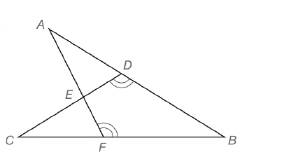Chapter 5.3, Problem 31EElementary Geometry For College St...

7th Edition
Alexander + 2 others
ISBN: 9781337614085

Solutions

Chapter
SectionElementary Geometry For College St...

7th Edition
Alexander + 2 others
ISBN: 9781337614085
Textbook Problem

Δ A B F ∼ Δ C B D with obtuse angles at vertices D and F as indicated. If m ∠ B = 45 ° , m ∠ C = x and m ∠ A F B = 4 x , find x.Exercises 31, 32

To determine

To find:

The value of x in degree by using the given information.

Explanation

Definition:

CASTC:

Corresponding angles of similar triangles are congruent.

Description:

Given that ΔABFΔCBD with obtuse angles at vertices D and F as indicated. If mB=45°, mC=x and mAFB=4x.

The given figure is shown below.

Figure 1

From the given figure, it is observed that there are two triangles ABF and CBD and which is given that two triangles are similar.

Now draw the two triangles separately as shown below.

Figure 2

Figure 3

Since ΔABFΔCBD and from the definition of CASTC, corresponding angles of similar triangles are congruent

Still sussing out bartleby?

Check out a sample textbook solution.

See a sample solution

The Solution to Your Study Problems

Bartleby provides explanations to thousands of textbook problems written by our experts, many with advanced degrees!

Get Started

Fill in each blank: 93ft=yd

Elementary Technical Mathematics

In Problems 33 – 38, solve each inequality. 38.

Mathematical Applications for the Management, Life, and Social Sciences

Which is the best graph of y = f (x) for the given graph of y = f (x)? a) None of these

Study Guide for Stewart's Single Variable Calculus: Early Transcendentals, 8th